# Graph, random

A probabilistic model for the study of frequency characteristics of various graph parameters. A random graph is usually understood to mean a certain class of graphs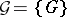with a given probability distribution. An arbitrary graphfromis said to be a realization of the random graph. Any numerical characteristic (parameter) of a graph (cf. Graph, numerical characteristics of a) can be regarded as a random variable. The concept of a random graph is highly useful in modelling connection networks which are subject to certain random changes or of logical networks whose elements may assume defective states; in considering a picture of phase transformations in statistical physics; in studying various biological processes; in problems of minimization of a Boolean function, etc. In several cases the concept of a random graph makes it possible to utilize probability theory as a tool in order to obtain asymptotic solutions of enumeration problems.

In a typical construction of a random graph, all realizations are obtained by applying to a non-random graph(most often a complete graph) a certain procedure for the removal of its edges; it is usually assumed in so doing that the removals of different edges are independent events, and that the removal of an edgeoccurs with probability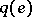. Such a construction of a random graph is denoted by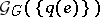. Of greatest interest is the study of the various numerical connectivity characteristics of a random graph, such as the number of connected components, the diameter, radius, the connectivity number, etc., which can be interpreted as characteristics of the reliability of the respective connection network or logical network. In such a case the numbercharacterizes the reliability of a connection, whileis the probability of its leaving the system.

Letbe a complete graph withvertices and let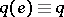,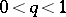. The random graphis connected, has diameter and radius equal to 2 and contains a Hamilton cycle (cf. Graph circuit) with a probability which tends to one astends to infinity. Ifis a function of the number of vertices, then the probability of the random graphbeing connected depends on the closeness ofto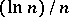. More exactly, let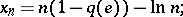then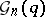tends to one as, if; it tends to zero if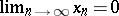; it tends toif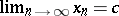, whereis a constant. In the last-mentioned case the number of edges of the random graph is asymptotically equal to.

The same random graphcan also be regarded as the graph obtained from the non-random empty graph withvertices by randomly connecting its vertices by edges so that any pair of vertices has probability of being connected, independently of the others, equal to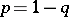. This mode of formation of the graphis made dynamic if one puts,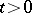,being assigned the meaning of time. The situation is then as follows. At the initial moment of time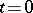one hasseparate vertices. Then, as the value ofincreases, there appear non-trivial connected components, representing trees or connected graphs with one cycle and a small number of vertices. Next there appears one "main" component containing a number of vertices asymptotically equal to(for large values of). The process is subsequently distinguished by growth of the main component and by decrease in the number of small components. Finally, at some moment of time, the graph becomes connected. This evolution of the random graph can be regarded as the model of the picture of phase transformations, where the main component plays the role of the liquid phase, while the part of the rarified phase is played by components with a small number of vertices.

There exist many other types of random graphs, e.g. those connected with trees (random trees), with one-place mappings of a finite set into itself (random mappings), and with subgraphs of the-dimensional unit cube (random Boolean functions).